# NCERT Solutions for Class 8 Maths Chapter 6 Exercise 6.3

NCERT Solutions for Class 8 Maths Chapter 6 Exercise 6.1 – Square and Square roots, has been designed by the NCERT to test the knowledge of the student on the topic – Square Roots

• Finding square roots
• Finding square root through repeated subtraction
• Finding square root through prime factorisation

###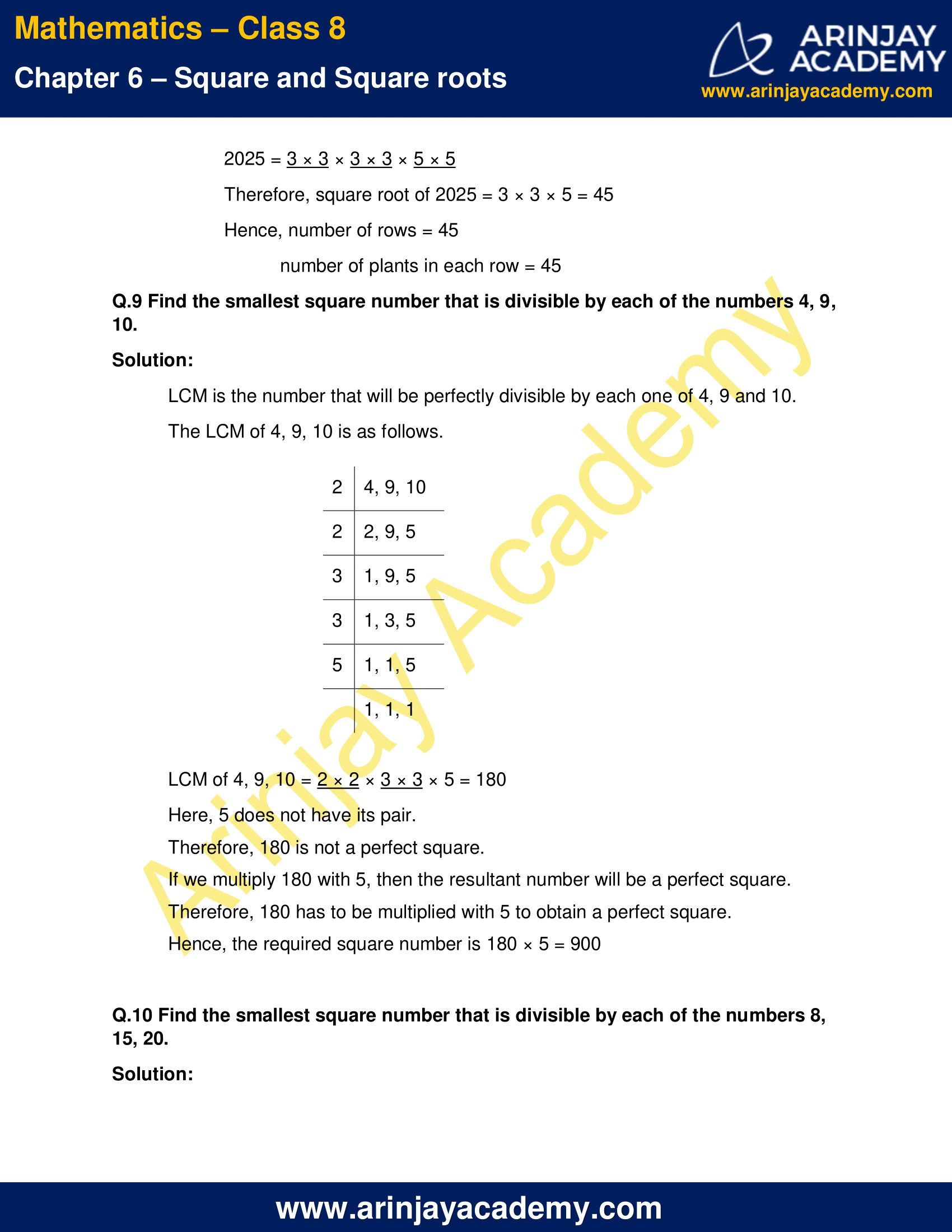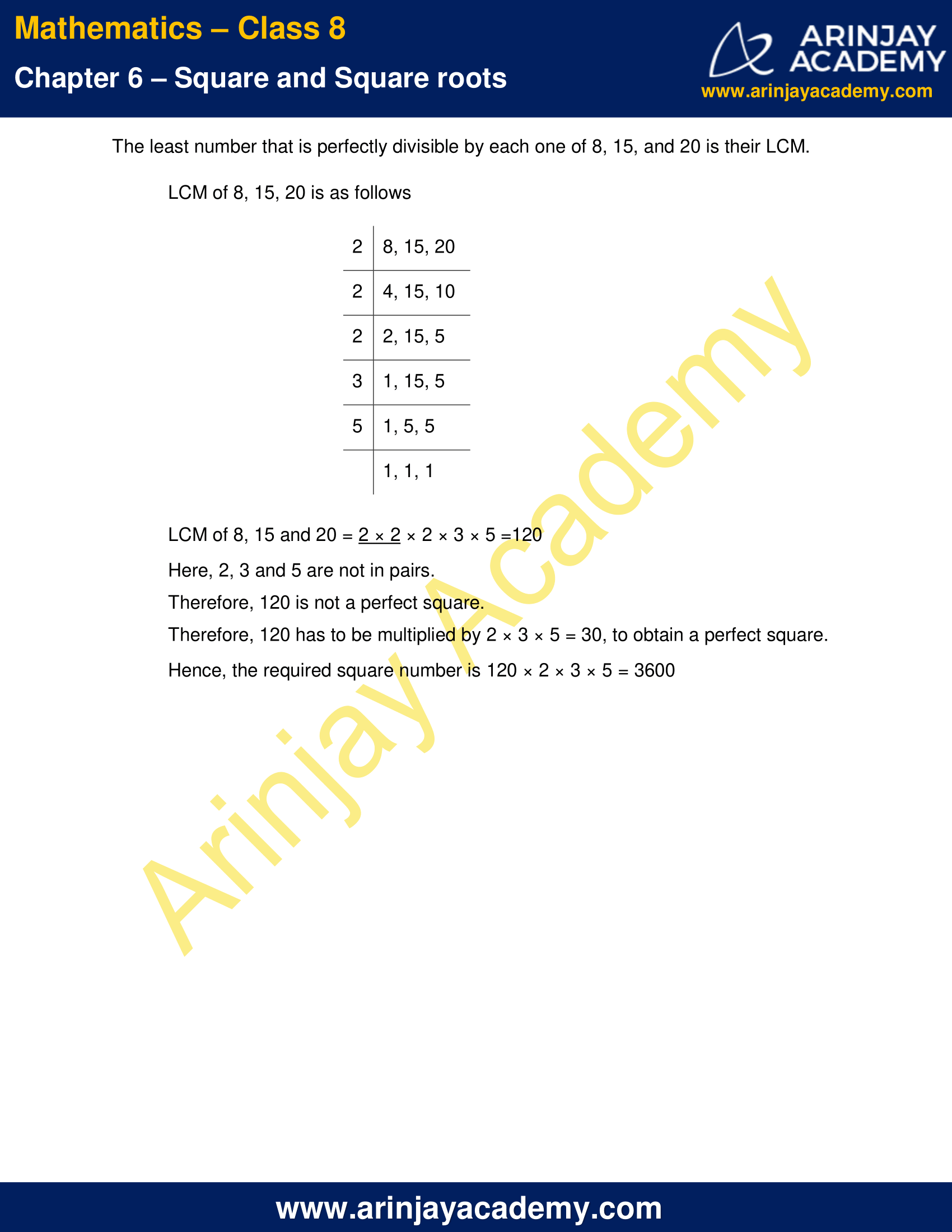NCERT Solutions for Class 8 Maths Chapter 6 Exercise 6.3

Q.1 What could be the possible ‘one’s ‘ digits of the square root of each of the following numbers?

(i) 9801
(ii) 99856
(iii) 998001
(iv) 657666025

Solution:

(i) As 9801 has 1 in the unit’s place, the one’s digit of its square root could be either 1 or 9
(ii) As 99856 has 6 in the unit’s place, the one’s digit of its square root could be either 4 or 6
(iii) As 998001 has 1 in the unit’s place, the one’s digit of its square root could be either 1 or 9
(iii) As 657666025 has 5 in the unit’s place, the one’s digit of its square root could be 5

Q.2 Without doing any calculation, find the numbers which are surely not perfect squares.

(i) 153
(ii) 257
(iii) 408
(iv) 441

Solution:

As we know, the perfect square of a number has the digits 0, 1, 4, 5, 6 or 9 at unit’s place. Also, a perfect square will end with even number of zeroes.

(i) 153 has 3 in unit’s place. Therefore 153 is not a perfect square
(ii) 257 has 7 in unit’s place. Therefore 257 is not a perfect square
(iii) 408 has 8 in unit’s place. Therefore 408 is not a perfect square
(iv) 441 has 1 in unit’s place. Therefore 441 can be a perfect square

Q.3 Find the square roots of 100 and 169 by the method of repeated subtraction.

Solution:

We know that, sum of first n odd natural numbers is n2. That is, every square number can be expressed as a sum of successive odd natural numbers from 1.

(i) Consider √100

(i) 100 − 1 = 99
(ii) 99 − 3 = 96
(iii) 96 − 5 = 91
(iv) 91 − 7 = 84
(v) 84 − 9 = 75
(vi) 75 − 11= 64
(vii) 64 − 13 = 51
(viii) 51 − 15 = 36
(ix) 36 − 17 = 19
(x) 19 − 19 = 0

From 100 we have subtracted successive odd numbers starting from 1 and obtained 0 at the 10th step.
Therefore, √100 = 10

(ii) Consider √169

(i) 169 − 1 = 168
(ii) 168 − 3 = 165
(iii) 165 − 5 = 160
(iv) 160 − 7 = 153
(v) 153 − 9 = 144
(vi) 144 − 11 = 133
(vii)133 − 13 = 120
(viii)120 – 15 =105
(ix) 105 − 17 = 88
(x) 88 − 19 = 69
(xi) 69 − 21 = 48
(xii) 48 − 23 = 25
(xiii)25 − 25 = 0

From 169 we have subtracted successive odd numbers starting from 1 and obtained 0 at the 13th step.
Therefore, √169 = 13

Q.4 Find the square roots of the following numbers by the Prime Factorisation Method.
(i) 729
(ii) 400
(iii) 1764
(iv) 4096
(v) 7744
(vi) 9604
(vii) 5929
(viii) 9216
(ix) 529
(x) 8100

(i) Prime Factorization of 729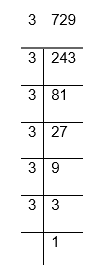729 = 3 × 3 × 3 × 3 × 3 × 3
Therefore, square root of 729 = 3 ×3 × 3 = 27

(ii) Prime Factorization of 400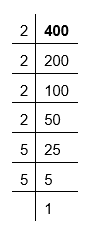400 = 2 × 2 × 2 × 2 × 5 × 5
Therefore, square root of 400 = 2 × 2 × 5 = 20

(iii) Prime Factorization of 1764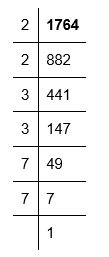1764 = 2 × 2 × 3 × 3 × 7 × 7
Therefore, Square root of 1764 = 2 × 3 × 7 = 42

(iv) Prime Factorization of 4096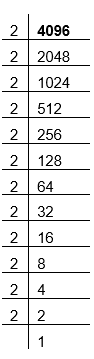4096 = 2 × 2 × 2 × 2 × 2 × 2 × 2 × 2 × 2 × 2 × 2 × 2
Therefore, square root of 4096 = 2 × 2 × 2 × 2 × 2 × 2 = 64

(v) Prime Factorization of 7744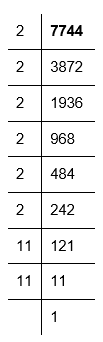7744 = 2 × 2 × 2 × 2 × 2 × 2 × 11 × 11
Therefore, square root of 7744 = 2 × 2 × 2 × 11 = 88

(vi) Prime Factorization of 9604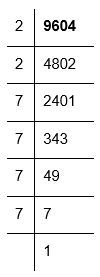9604 = 2 × 2 × 7 × 7 × 7 × 7
Therefore, square root of 9604 = 2 × 7 × 7 = 98

(vii) Prime Factorization of 5929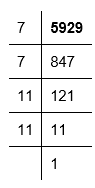5929 = 7 × 7 × 11 × 11
Therefore, square root of 5929 = 7 × 11 = 77

(viii) Prime Factorization of 9216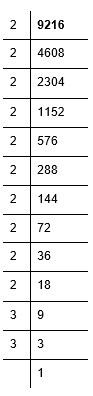9216 = 2 × 2 × 2 × 2 × 2 × 2 × 2 × 2 × 2 × 2 × 3 × 3
Therefore, square root of 9216 = 2 × 2 × 2 × 2 × 2 × 3 = 96

(ix) Prime Factorization of 529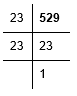529 = 23 × 23
Therefore, Square root of 529 = 23

(x) Prime Factorization of 8100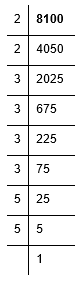8100 = 2 × 2 × 3 × 3 × 3 × 3 × 5 × 5
Therefore, square root of 8100 = 2×3×3×5 = 90

Q.5 For each of the following numbers, find the smallest whole number by which it should be multiplied so as to get a perfect square number. Also find the square root of the square number so obtained.

(i) 252
(ii) 180
(iii) 1008
(iv) 2028
(v) 1458
(vi) 768

Solution:

(i) Prime factorization of 252 is as follows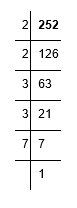252 = 2 × 2 × 3 × 3 × 7
Here, 7 does not have its pair.
Therefore, 252 has to be multiplied with 7 to obtain a perfect square.
252 × 7 = 2 × 2 × 3 × 3 × 7 × 7
Therefore, 252 × 7 = 1764 is a perfect square.
Square root of 1764 = 2 × 3 × 7 = 42

(ii) Prime factorization of 180 is as follows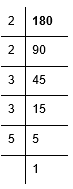180 = 2 × 2 × 3 × 3 × 5
Here, 5 does not have its pair
Therefore, 180 has to be multiplied with 5 to make it a perfect square.
180 × 5 = 2 × 2 × 3 × 3 × 5 × 5
Therefore, 180 × 5 = 900 is a perfect square
Square root of 900 = 2 × 3 × 5 = 30

(iii) Prime factorization of 1008 is as follows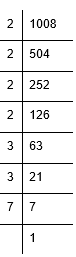1008 = 2 × 2 × 2 × 2 × 3 × 3 × 7
Here, among the factors of 1008, 7 does not have its pair
Therefore, 1008 has to be multiplied with 7 to make it a perfect square.
1008 × 7 = 2 × 2 × 2 × 2 × 3 × 3 × 7 × 7
Therefore, 1008 × 7 = 7056 is a perfect square
Square root of 7056 = 2 × 2 × 3 × 7 = 84

(iv) Prime factorization of 2028 is as follows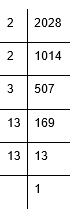2028 = 2 × 2 × 3 × 13 × 13
Here, among the factors of 2028, 3 does not have its pair
Therefore, 2028 has to be multiplied with 3 to make it a perfect square.
2028 × 3 = 2 × 2 × 3 × 3 × 13 × 13
Therefore, 2028 × 3 = 6084 is a perfect square
Square root of 6084 = 2 × 3 × 13 = 78

(v) Prime factorization of 1458 is as follows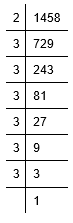1458 = 2 × 3 × 3 × 3 × 3 × 3 × 3
Here, among the factors of 1458, 2 does not have its pair
Therefore, 1458 has to be multiplied with 2 to make it a perfect square.
1458 = 2 × 2 × 3 × 3 × 3 × 3 × 3 × 3
Therefore, 1458 × 2 = 2916 is a perfect square
Square root of 2916 = 2 × 3 × 3 × 3 = 54

(vi) Prime factorization of 768 is as follows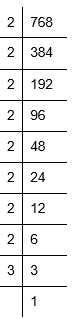768 = 2 × 2 × 2 × 2 × 2 × 2 × 2 × 2 × 3
Here, among the factors of 768, 3 does not have its pair
Therefore, 768 has to be multiplied with 3 to make it a perfect square.
768 × 3 = 2 × 2 × 2 × 2 × 2 × 2 × 2 × 2 × 3 × 3
Therefore, 768 × 3 = 2304 is a perfect square
Square root of 2304 = 2 × 2 × 2 × 2 × 3 = 48

Q.6 For each of the following numbers, find the smallest whole number by which it should be divided so as to get a perfect square number. Also find the square root of the square number so obtained.

(i) 252
(ii) 2925
(iii) 396
(iv) 2645
(v) 2800
(vi) 1620

Solution:

(i) Prime factorization of 252 is as follows.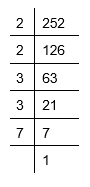252 = 2 × 2 × 3 × 3 × 7
Here, 7 does not have its pair.
If we divide 252 by 7, then the number will become a perfect square.
Therefore, 252 has to be divided by 7 to obtain a perfect square.
252 ÷ 7 = 36 is a perfect square.
36 = 2 × 2 × 3 × 3
Therefore, square root of 36 = 2 × 3 = 6

(ii) Prime factorization of 2925 is as follows.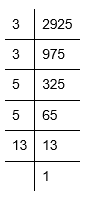2925 = 3 × 3 × 5 × 5 × 13
Here, 13 does not have its pair.
If we divide 2925 by 13, then the resultant number will become a perfect square.
Therefore, 2925 has to be divided by 13 to obtain a perfect square.
2925 ÷ 13 = 225 is a perfect square.
225 = 3 × 3 × 5 × 5
Therefore, square root of 225 = 3 × 5 = 15

(iii) Prime factorization of 396 is as follows.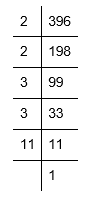396 = 2 × 2 × 3 × 3 × 11
Here, 11 does not have its pair.
If we divide 396 by 11, then the resultant number will become a perfect square.
Therefore, 396 has to be divided by 11 to obtain a perfect square.
396 ÷ 11 = 36 is a perfect square.
36 =  2 × 2 × 3 × 3
Therefore, square root of 36 = 2 × 3 = 6

(iv) Prime factorization of 2645 is as follows.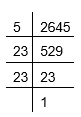2645 = 5 × 23 × 23
Here, 5 does not have its pair.
If we divide 2645 by 5, then the resultant number will become a perfect square.
Therefore, 2645 has to be divided by 5 to obtain a perfect square.
2645 ÷5 = 529 is a perfect square.
529 = 23 × 23
Therefore, square root of 529 = 23

(v) Prime factorization of 2800 is as follows.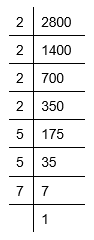2800 = 2 × 2 × 2 × 2 × 5 × 5 × 7
Here, prime factor 7 does not have its pair.
If we divide 700 by 7, then the resultant number will become a perfect square.
Therefore, 2800 has to be divided by 7 to obtain a perfect square.
2800 ÷7 = 400 is a perfect square.
400 = 2 × 2 × 2 × 2 × 5 × 5
Therefore, square root of 400 = 2 × 2 × 5 = 20

(vi) 1620 can be factorised as follows.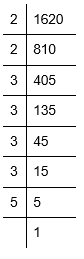1620 = 2 × 2 × 3 × 3 × 3 × 3 × 5
Here, 5 does not have its pair.
If we divide 1620 by 5, then the resultant number will become a perfect square.
Therefore, 1620 has to be divided by 5 to obtain a perfect square.
1620 ÷5 = 324 is a perfect square.
324 = 2 × 2 × 3 × 3 × 3 × 3
Therefore, square root of 324 = 2 × 3 × 3 = 18

Q.7 The students of Class VIII of a school donated Rs 2401 in all, for Prime Minister’s National Relief Fund. Each student donated as many rupees as the number of students in the class. Find the number of students in the class.

Solution:

Given,
Students of Class VIII of a school donated Rs 2401 in all, for Prime Minister’s National Relief Fund.
Each student donated as many rupees as the number of students in the class

Therefore,
Number of students in the class = Money donated by each student = √2401
Prime Factorization of 2401 is as follows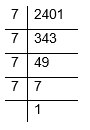2401 = 7 × 7 × 7 × 7
Therefore, square root of 2401 = 7 × 7 = 49
Hence, number of students in the class = 49

Q.8 2025 plants are to be planted in a garden in such a way that each row contains as many plants as the number of rows. Find the number of rows and the number of plants in each row.

Solution:

Given,
2025 plants are to be planted in a garden in such a way that each row contains as many plants as the number of rows.
Number of rows = number of plants in each row = √2025
Prime Factorization of 2025 is as follows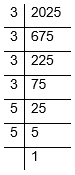2025 = 3 × 3 × 3 × 3 × 5 × 5
Therefore, square root of 2025 = 3 × 3 × 5 = 45
Hence, number of rows = 45
Number of plants in each row = 45

Q.9 Find the smallest square number that is divisible by each of the numbers 4, 9, 10.

Solution:

LCM is the number that will be perfectly divisible by each one of 4, 9 and 10.
The LCM of 4, 9, 10 is as follows.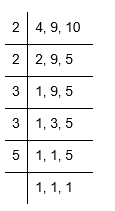LCM of 4, 9, 10 = 2 × 2 × 3 × 3 × 5 = 180
Here, 5 does not have its pair.
Therefore, 180 is not a perfect square.
If we multiply 180 with 5, then the resultant number will be a perfect square. Therefore, 180 has to be multiplied with 5 to obtain a perfect square.
Hence, the required square number is 180 × 5 = 900

Q.10 Find the smallest square number that is divisible by each of the numbers 8, 15, 20.

Solution:

The least number that is perfectly divisible by each one of 8, 15, and 20 is their LCM.

LCM of 8, 15, 20 is as follows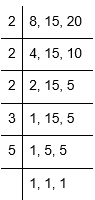LCM of 8, 15 and 20 = 2 × 2 × 2 × 3 × 5 =120
Here, 2, 3 and 5 are not in pairs.
Therefore, 120 is not a perfect square.
Therefore, 120 has to be multiplied by 2 × 3 × 5 = 30, to obtain a perfect square.
Hence, the required square number is 120 × 2 × 3 × 5 = 3600

The next Exercise for NCERT Solutions for Class 8 Maths Chapter 6 Exercise 6.4 – Square and Square roots can be accessed by clicking here